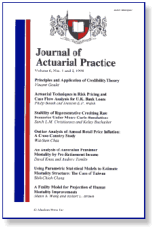Finance DepartmentJournal of Actuarial Practice 1993-2006

2005

Article

Citation

Journal of Actuarial Practice 12 (2005), pp. 159-180

Copyright 2005 Absalom Press

Abstract

The paper investigates the individual aggregate cost method (also known as the individual spread-gain method), which is normally applicable in small pension funds or fully contributory schemes, using a control theoretical framework. We construct the difference equations describing the mechanisms of the respective funding method and then calculate the optimal control path of the contribution rate assuming (first) a stochastic and (second) a deterministic pattern for the future investment rates of return. For the first case, the optimal solution is achieved through a linear approximation and using stochastic optimization techniques. It is proved that the contribution rate is (optimally) controlled through the control of the valuation rate (which is determined incorporating a certain feedback mechanism of the past contribution rate). The optimal solution for the deterministic case is obtained using standard calculus and the method of Lagrange multipliers.

COinS